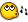## Recommended Posts

hi all,

following script is supposed to sort the elements of excel sheet column.

```Dim \$excel=ObjCreate("excel.application")
\$excel.visible=1

\$excel.ActiveWorkBook.ActiveSheet.Cells(1,1).Value="hello"         ; Fill a cell
\$excel.ActiveWorkBook.ActiveSheet.Cells(2,1).Value="Cello"        ; Fill a cell
\$excel.ActiveWorkBook.ActiveSheet.Cells(3,1).Value="Dello"        ; Fill a cell
\$excel.ActiveWorkBook.ActiveSheet.Cells(4,1).Value="Aello"        ; Fill a cell
\$excel.ActiveWorkBook.ActiveSheet.Cells(5,1).Value="Bello"        ; Fill a cell

\$excel.ActiveWorkBook.ActiveSheet.Range("A1:A5").Select.Sort("A")

\$excel.ActiveWorkBook.Saved = 1```

##### Share on other sites

Hello neotrio,

Try this:

```Dim \$excel=ObjCreate("excel.application")
\$excel.visible=1

\$excel.ActiveWorkBook.ActiveSheet.Range("A1:A5").Select
\$excel.ActiveWorkBook.ActiveSheet.Cells(1,1).Value="hello"; Fill a cell
\$excel.ActiveWorkBook.ActiveSheet.Cells(2,1).Value="Cello"; Fill a cell
\$excel.ActiveWorkBook.ActiveSheet.Cells(3,1).Value="Dello"; Fill a cell
\$excel.ActiveWorkBook.ActiveSheet.Cells(4,1).Value="Aello"; Fill a cell
\$excel.ActiveWorkBook.ActiveSheet.Cells(5,1).Value="Bello"; Fill a cell

\$excel.ActiveWorkBook.ActiveSheet.Range("A1:A5").Select
\$Key = \$excel.ActiveWorkBook.ActiveSheet.Cells(1,1)
\$excel.ActiveWorkBook.ActiveSheet.Range("A1:A5").Sort(\$Key)

\$excel.ActiveWorkBook.Saved = 1```

##### Share on other sites

Thank you, zfisherdrums.Hello neotrio,

Try this:

```Dim \$excel=ObjCreate("excel.application")
\$excel.visible=1
\$excel.ActiveWorkBook.ActiveSheet.Range("A1:A5").Select
\$excel.ActiveWorkBook.ActiveSheet.Cells(1,1).Value="hello"; Fill a cell
\$excel.ActiveWorkBook.ActiveSheet.Cells(2,1).Value="Cello"; Fill a cell
\$excel.ActiveWorkBook.ActiveSheet.Cells(3,1).Value="Dello"; Fill a cell
\$excel.ActiveWorkBook.ActiveSheet.Cells(4,1).Value="Aello"; Fill a cell
\$excel.ActiveWorkBook.ActiveSheet.Cells(5,1).Value="Bello"; Fill a cell

\$excel.ActiveWorkBook.ActiveSheet.Range("A1:A5").Select
\$Key = \$excel.ActiveWorkBook.ActiveSheet.Cells(1,1)
\$excel.ActiveWorkBook.ActiveSheet.Range("A1:A5").Sort(\$Key)

\$excel.ActiveWorkBook.Saved = 1```

## Create an account

Register a new account

• ### Recently Browsing   0 members

×

• Wiki

• Back

• #### Beta

• Git
• FAQ
• Our Picks
×
• Create New...